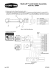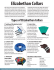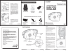# Homework 2

## Transcription

Homework 2
```Homework 2
1. Determine the force P required to
maintain the 200-kg engine in the
position for which q = 30o. The diameter
of the pulley at B is negligible.
2. A scale is constructed using the 10 kg
mass A, the 2 kg pan P and the pulley and
cord arrangement. Cord BCA is 2 m long. If
s = 0.75 m, determine the mass D in the
pan. Neglect the size of the pulley.
3. Determine the mass of each of the two
cylinders if they cause a sag of s = 0.5 m
when supported from the rings at A and
B. Note that s = 0 when the cylinders are
removed.
4. Determine the tensions in cables AB,
z
y
x
5. If the maximum allowable tension
in cables AB and AC is 2000 N,
determine the maximum height z to
which the 800 N crate can
 be lifted.
What horizontal force F must be
applied?
z
2m
B
2m
C
A
1.5 m
3m
z
x

F
y
W=800 N
6. Determine the stretch in each of the two
springs required to hold the 20 kg crate in
the equilibrium position shown. Each
spring has an unstretched length of 2 m
and a stiffness of k = 300 N/m.
7. Collar A weighing 170 N, which can
slide freely on the quarter circle, is held
in equilibrium by cable AB.
a) Determine the tension in the cable.
b) Determine the magnitude and the
components of the contact force acting
on the collar from the circle.
4.5 m
3.4 m
15
r =3.4 m
8
8. Straight bar AB is fixed in space. Spring CD
has a stiffness of 3 N/mm and its unstretched
length is 200 mm. If there is no friction between
collar C and bar AB, determine the weight W of
the collar that produces the equilibrium
condition shown and the reaction between the
collar and bar AB.
```

### lion country supply - The American Beagler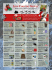### ROGZ UTILITY Landing Strip Collars 1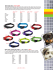### 1D Dress for Success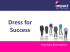### technician shirt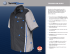### classic solid auto shirt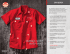### SOLAS Fireguard - P G Products Ltd.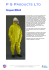### Document 6457942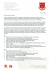### MF1494D MULTI-LIFTÂ® Limit Switch Assembly 39464 - Chore